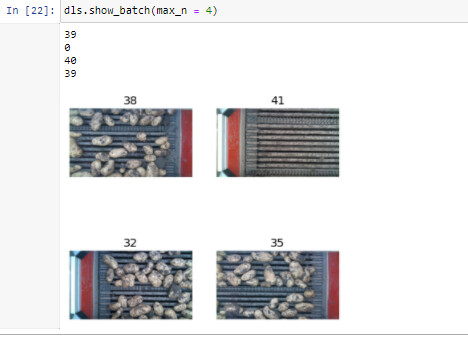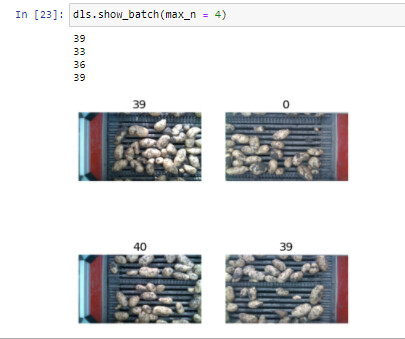# Show_batch() function does not show the correct labels

My goal is to predict the amount of potatoes in an image. I have put my labels in the image filenames and loaded them as follows:
"
path = Path(r"C:\Users\Kees\Desktop\WUR stage\Dataset\Test")
Path.BASE_PATH = path

def label_func(fname):
pattern = re.compile(r"(\d+).png")
matches= pattern.finditer(str(fname))
for match in matches:
x = match.group(1)
x = int(x)
print(x)
return x

cat_names = list(range(200))

potatoes = DataBlock(blocks = (ImageBlock, CategoryBlock),
get_items=get_image_files,
splitter=RandomSplitter(seed=42, valid_pct=0.05),
get_y=label_func,
batch_tfms = [Normalize.from_stats(*imagenet_stats)]
)

dls = potatoes.dataloaders(path,num_workers=0, bs=1,cat_names=cat_names)
"

I used a category block here but a regressionblock is probably more suited. Now when I use the show_batch function it does not show the correct labels above the pictures. I have created my own label function so that I can print out what the label is supposed to be.Everytime I run it the previous correct labels show up on the next batch:When I train the model it doesn’t learn at all so I think this also happen when I train. When I look at the .summary it takes the correct label from the filename and stores it with the correct image tensor:
"
Setting-up type transforms pipelines
Collecting items from C:\Users\Kees\Desktop\WUR stage\Dataset\Test
Found 1377 items
2 datasets of sizes 1309,68
Setting up Pipeline: PILBase.create
Setting up Pipeline: label_func -> Categorize – {‘vocab’: None, ‘sort’: True, ‘add_na’: False}
44

Building one sample
Pipeline: PILBase.create
starting from
C:\Users\Kees\Desktop\WUR stage\Dataset\Test\img_679_rgb0_44.png
applying PILBase.create gives
PILImage mode=RGB size=1280x720
Pipeline: label_func -> Categorize – {‘vocab’: None, ‘sort’: True, ‘add_na’: False}
starting from
C:\Users\Kees\Desktop\WUR stage\Dataset\Test\img_679_rgb0_44.png
44
applying label_func gives
44
applying Categorize – {‘vocab’: None, ‘sort’: True, ‘add_na’: False} gives
TensorCategory(44, dtype=torch.int32)
44

Final sample: (PILImage mode=RGB size=1280x720, TensorCategory(44, dtype=torch.int32))

Collecting items from C:\Users\Kees\Desktop\WUR stage\Dataset\Test
Found 1377 items
2 datasets of sizes 1309,68
Setting up Pipeline: PILBase.create
Setting up Pipeline: label_func -> Categorize – {‘vocab’: None, ‘sort’: True, ‘add_na’: False}
44
Setting up after_item: Pipeline: ToTensor
Setting up before_batch: Pipeline:
Setting up after_batch: Pipeline: IntToFloatTensor – {‘div’: 255.0, ‘div_mask’: 1} -> Normalize – {‘mean’: tensor([[[[0.4850]],

``````     [[0.4560]],

[[0.4060]]]], device='cuda:0'), 'std': tensor([[[[0.2290]],

[[0.2240]],

[[0.2250]]]], device='cuda:0'), 'axes': (0, 2, 3)}
``````

44

Building one batch
Applying item_tfms to the first sample:
44
Pipeline: ToTensor
starting from
(PILImage mode=RGB size=1280x720, TensorCategory(44, dtype=torch.int32))
applying ToTensor gives
(TensorImage of size 3x720x1280, TensorCategory(44, dtype=torch.int32))

Adding the next 3 samples
33
36
39

No before_batch transform to apply

Collating items in a batch

Applying batch_tfms to the batch built
Pipeline: IntToFloatTensor – {‘div’: 255.0, ‘div_mask’: 1} -> Normalize – {‘mean’: tensor([[[[0.4850]],

``````     [[0.4560]],

[[0.4060]]]], device='cuda:0'), 'std': tensor([[[[0.2290]],

[[0.2240]],

[[0.2250]]]], device='cuda:0'), 'axes': (0, 2, 3)}
starting from
(TensorImage of size 4x3x720x1280, TensorCategory([44, 33, 36, 39], device='cuda:0', dtype=torch.int32))
applying IntToFloatTensor -- {'div': 255.0, 'div_mask': 1} gives
(TensorImage of size 4x3x720x1280, TensorCategory([44, 33, 36, 39], device='cuda:0', dtype=torch.int32))
applying Normalize -- {'mean': tensor([[[[0.4850]],

[[0.4560]],

[[0.4060]]]], device='cuda:0'), 'std': tensor([[[[0.2290]],

[[0.2240]],

[[0.2250]]]], device='cuda:0'), 'axes': (0, 2, 3)} gives
(TensorImage of size 4x3x720x1280, TensorCategory([44, 33, 36, 39], device='cuda:0', dtype=torch.int32))
``````

"

Does anybody now what is going wrong? And how to fix it?
Thanks in advance,
Kees Geerligs

Blockquote